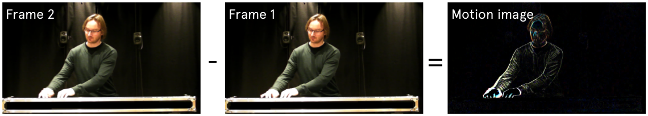# Feature Extraction

Several features can be extracted from a video file.## What is a video file?

Let us start by understanding more about what a video file contains. Find a video file on your computer and look at its metadata. On most systems (Linux, Mac, Windows), you should see some basic information about the video content by selecting something like “properties” from the file inspector (see example below).From this information, you should be able to answer these questions:

• What are the dimensions?
• What is the framerate?
• What type of compression is used?

All of these questions can be answered by looking at the metadata of the file. From the description of our example video above, we can see that the dimensions are 1920 x 1080 pixels. This is one of the most common video formats and is a so-called “landscape” image in a full HD resolution. The video file dimensions are important because it tells something about how much information there is in the file and how much data there is to process.

From the information about the file, we can also see that the framerate is 29.97 frames per second (fps). Usually, video frame rates are either 25, 29.97, or 30 fps, but they can also be higher. The frame rate influences how fast motion we can analyse. In many cases, a standard frame rate is sufficient. But for rapid motion, one would need a higher frame rate to capture the action.

## Video as a stream of numbers

A digital video file is a collection of numbers, as illustrated in the figure below. In a typical video file, each pixel is stored 8-bit resolution. That means that a number between 0 and 255 represents each pixel, where 0 means black and 255 means white. A video file is a series of matrices with numbers. Each of the matrices contains four planes.Also, video files in colour have four planes per pixel: the alpha channel (transparency), red, green, and blue. A greyscale video only has one plane per pixel. So greyscale files are 1/4 the size of a colour file. Similarly, processing greyscale files take 1/4 of the time of a colour file. This is important to remember when doing computational video analysis.

## Quantity of motion

One of the simplest motion features we can extract from a video file can be called the quantity of motion. This can be calculated by summing up all the “active” pixels in a motion image. If you recall from the previous article, a motion image shows the differences between frames.In the image above, you see the changes as an image, but this image could also have been represented with numbers. Each of the black pixels has a value of 0, while white pixels have a value of 255 (the maximum value of an 8-bit file). Grey pixels will have a number somewhere between 0 and 255. We can then sum up all the image values to get the total number of active pixels in the image. Since images may be of different sizes, we often normalize the number in the end. This is done by dividing the total active pixels number by the number of pixels in the image. This will give us a number that is somewhere between 0 and 1. This is then the “quantity of motion” of that frame.

Plotting the data in a graph can give something like this:This value is a rough estimate of the activity going on, but it may be sufficient in many cases. It is a very lightweight calculation in comparison to other, more advanced computer vision algorithms. Thus it is useful for real-time applications.

## Aread and Centroid of Motion

Some other basic features that can be extracted from a motion image are the Area of Motion (AoM) and Centroid of Motion (CoM). These should not be confused with the are and centre of the body, as illustrated in the figure below.While the QoM tells about how much activity there is an image sequence, the AoM and CoM reflect where in the image the activity is happening.

## Pose Estimation

The above techniques are among the easiest ones to implement. There are numerous more advanced methods for extracting all sorts of things from video files. This includes tracking objects and faces.

With recent machine learning-based computer vision models, it is even possible to extract information about multiple body landmarks and how they move over time.This is a powerful type of analysis but also computationally heavy. As can be seen from the image above, the tracking accuracy is much lower than what you find with the infrared motion capture systems. Still, such a video-based pose estimation may be sufficient for many needs.